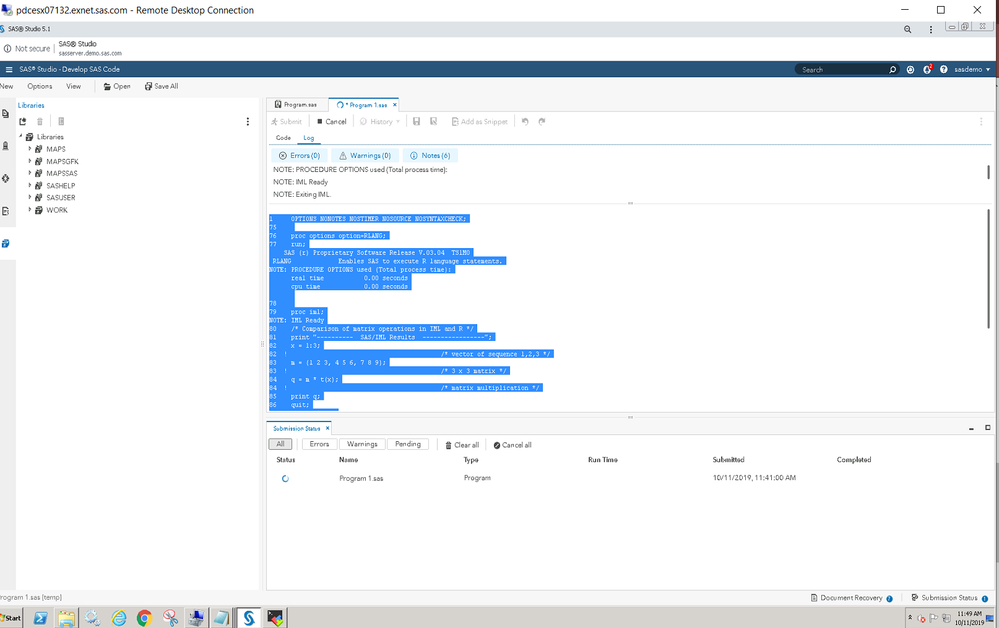## Submit/R and R statements in PROC IML is running very slowly (On SAS Viya Studio 4.1 and 5)

My RLANG System Option has already determined that I am able to call R from SAS System.

I have also set up R_HOME environment variable that points to the correct directory that contains LibR.so. This has been correctly configured on the config file that RLANG System Option value stated.

These are the codes that I have submitted on the studio (I tried both):

```proc options option=RLANG value;
run;

options set=R_HOME='/opt/anaconda3/lib/R';
%put %sysget(R_HOME);

proc iml;
/* Comparison of matrix operations in IML and R */
print "----------  SAS/IML Results  -----------------";
x = 1:3;                                 /* vector of sequence 1,2,3 */
m = {1 2 3, 4 5 6, 7 8 9};               /* 3 x 3 matrix */
q = m * t(x);                            /* matrix multiplication */
print q;
print "-------------  R Results  --------------------";
submit / R;
rx <- matrix( 1:3, nrow=1);             # vector of sequence 1,2,3
rm <- matrix( 1:9, nrow=3, byrow=TRUE); # 3 x 3 matrix
rq <- rm %*% t(rx);                     # matrix multiplication
print(rq);
endsubmit;
quit;```

While my result for SAS/IML successfully displayed on output, the moment I placed the R results code in, the program keeps being on running mode for long hours and it is on loop. Is there any way to resolve this issue since there is no error log found and the settings were done correctly?

2 REPLIES 2

## Re: Submit/R and R statements in PROC IML is running very slowly (On SAS Viya Studio 4.1 and 5)

Did you try running the two bits of code as separate calls to proc Iml?

```proc iml;
/* Comparison of matrix operations in IML and R */
print "----------  SAS/IML Results  -----------------";
x = 1:3;                                 /* vector of sequence 1,2,3 */
m = {1 2 3, 4 5 6, 7 8 9};               /* 3 x 3 matrix */
q = m * t(x);                            /* matrix multiplication */
print q;

quit;

proc iml;
print "-------------  R Results  --------------------";
submit / R;
rx <- matrix( 1:3, nrow=1);             # vector of sequence 1,2,3
rm <- matrix( 1:9, nrow=3, byrow=TRUE); # 3 x 3 matrix
rq <- rm %*% t(rx);                     # matrix multiplication
print(rq);
endsubmit;
quit;```

## Re: Submit/R and R statements in PROC IML is running very slowly (On SAS Viya Studio 4.1 and 5)

Hi there,

I managed to run the two bits of code as separate calls to proc iml. I was able to get real time and cpu time of the SAS/IML Results, but not R Results. This is what I have on the log so far when I run the whole code.

```1     OPTIONS NONOTES NOSTIMER NOSOURCE NOSYNTAXCHECK;
75
76    proc options option=RLANG;
77    run;
SAS (r) Proprietary Software Release V.03.04  TS1M0
RLANG             Enables SAS to execute R language statements.
NOTE: PROCEDURE OPTIONS used (Total process time):
real time           0.00 seconds
cpu time            0.00 seconds

78
79    proc iml;
80    /* Comparison of matrix operations in IML and R */
81    print "----------  SAS/IML Results  -----------------";
82    x = 1:3;
82  !                                          /* vector of sequence 1,2,3 */
83    m = {1 2 3, 4 5 6, 7 8 9};
83  !                                          /* 3 x 3 matrix */
84    q = m * t(x);
84  !                                          /* matrix multiplication */
85    print q;
86    quit;
NOTE: Exiting IML.
NOTE: The PROCEDURE IML printed page 1.
NOTE: PROCEDURE IML used (Total process time):
real time           0.01 seconds
cpu time            0.01 seconds

87
88    proc iml;
89    print "-------------  R Results  --------------------";
90    submit / R;
91      rx <- matrix( 1:3, nrow=1);             # vector of sequence 1,2,3
92      rm <- matrix( 1:9, nrow=3, byrow=TRUE); # 3 x 3 matrix
93      rq <- rm %*% t(rx);                     # matrix multiplication
94      print(rq);
95    endsubmit;```

As you can tell, the SAS Studio is just running the code for R Results without any error nor completion and it has been like this for more than an hour. This is how it looks like currently after I submitted the two bits of code on SAS Studio V. It just keeps running.From The DO Loop